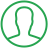# Python Histogram From List

Last Updated : Oct 16, 2023In this article we will show you the solution of python histogram from list, for engineers, histograms are an important sort of statistical plot. When compared to a set of value ranges, a histogram displays the frequency or number of values.

Python and the graphing software matplotlib can be used to make histogram charts.

Plotting a histogram is done using the plt.hist() method. Other programmers may be accustomed to the alias plt since it is frequently used to refer to the pyplot library by matplotlib.

We'll also import Numpy in our example using the command import Numpy as np.

We'll construct a dataset for plotting by using the random number generator in numpy.

Include the line%matplotlib inline below the imports if using a Jupyter notebook.

We will now discuss the idea of how to create a histogram from a list in python with an example.

## Step By Step Guide On Python Histogram From List :-

```import numpy as np
for s in ['bottom', 'top', 'right', 'left']:
axis.spines[s].set_visible(False)
axis.xaxis.set_ticks_position('none')
axis.yaxis.set_ticks_position('none')
axis.grid(visible = True, color = 'pink',
linestyle = '-.', linewidth = 0.6,
alpha = 0.5)
figure.text(1.0, 0.16, 'TalkersCode',
fontsize = 10,
color ='grey',
ha ='right',
alpha = 1.0)
N, bins, patche = axis.hist(x_axis, bins = no_bins)
frac = ((N**(1 / 5)) / N.max())
normal = colors.Normalize(frac.min(), frac.max())
for f, s in zip(frac, patche):
color = plt.cm.magma(normal(f))
s.set_facecolor(color)
plt.xlabel("X-axis")
plt.ylabel("Y-axis")
plt.title('Modified histogram')
plt.show()```
1. Import the necessary libraries, including colors from Matplotlib, PercentFormatter from Matplotlib, and Matplotlib.pyplot.
2. The axis' splines can be eliminated using a for loop.
3. Take off the axes' x and y ticks.
4. Set a space between the labels and axis.
5. Add gridlines for x and y.
6. Utilize the text() function of the Figure object to add a text watermark.
7. The hist() function can be used to create a histogram by giving the x-axis data and the number of bins as arguments.
8. Using the Normalize function from colors, change the color of the histogram columns according to the frequency.
9. The facecolor of each patch is set using the magma colormap when you iterate through the frequencies and patches of the histogram columns.
10. The histogram should have labels and a title.
11. Use the show() function to display the plot.

## Conclusion :-

As a result, we have successfully learned how to create a histogram from a list in python with an example.

In this article, we used Python and the Matplotlib plotting library to create two histograms.

An assortment of random numbers with a normal distribution were included in the first histogram.

I hope this article on python histogram from list helps you and the steps and method mentioned above are easy to follow and implement.

##About Pragati

Experienced coding content writer who enjoys breaking down complex concepts in programming languages like Java, Python, C, and C++. Over three years of experience producing interesting and relevant content for a variety of entities. Committed to providing concise and easy-to-understand articles that assist readers in easily understanding technology and industry trends in the world of coding and software development.